upm  1.7.1 Sensor/Actuator repository for libmraa (v2.0.0)
NRF24L01 Class Reference

API for the NRF24L01 Transceiver Module. More...

## Detailed Description

id This module defines the NRF24L01 interface for libnrf24l01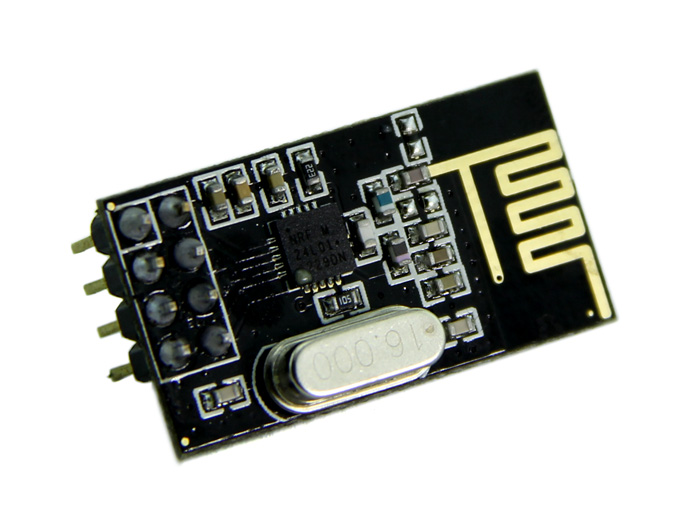class mycb : public virtual Callback
{
public:
mycb(upm::NRF24L01 *com) : _com(com) {}
virtual void run()
{
if (_com != NULL)
std::cout << "Reciever :: " << *((uint32_t*) &(_com->m_rxBuffer)) << std::endl;
else
std::cout << "Example callback!" << std::endl;
}
private:
};
int
main(int argc, char** argv)
{
mycb cb(&comm);
comm.configure();
comm.setSpeedRate(upm::NRF_250KBPS);
comm.setChannel(99);
signal(SIGINT, sig_handler);
while (!running) {
comm.pollListener();
}
std::cout << "exiting application" << std::endl;
return 0;
}
uint32_t dummyData = 0;
upm::NRF24L01 comm(7, 8);
mycb cb(&comm);
comm.setChannel(99);
comm.configure();
signal(SIGINT, sig_handler);
while (!running) {
memcpy(comm.m_txBuffer, &dummyData, sizeof(dummyData));
comm.send();
std::cout << "devi2 :: sending data ...." << dummyData << std::endl;
upm_delay_us(3000000);
dummyData += 3000;
}
std::cout << "exiting application" << std::endl;
upm::NRF24L01 sensor(7, 8);
sensor.setBeaconingMode();
std::vector<std::string> msgs;
msgs.push_back("Hello World 1!!!");
msgs.push_back("Hello World 2!!!");
msgs.push_back("Hello World 3!!!");
msgs.push_back("Hello World 4!!!");
msgs.push_back("Hello World 5!!!");
signal(SIGINT, sig_handler);
while (!running) {
for (std::vector<std::string>::iterator item = msgs.begin(); item != msgs.end(); ++item) {
std::cout << "BROADCASTING " << (*item).c_str() << std::endl;
for (int i = 0; i < 3; i++) {
sensor.sendBeaconingMsg((uint8_t*) (*item).c_str());
upm_delay_us(1000000);
}
}
}
std::cout << "exiting application" << std::endl;
msgs.clear();

## Public Member Functions

NRF24L01 (int cs, int ce)

std::string name ()

void init (int chipSelect, int chipEnable)

void configure ()

void send (uint8_t *value)

void send ()

void setDataReceivedHandler (Callback *call_obj)

bool dataSending ()

void getData (uint8_t *data)

uint8_t getStatus ()

bool rxFifoEmpty ()

void rxPowerUp ()

void rxFlushBuffer ()

void txPowerUp ()

void powerDown ()

void setChannel (uint8_t channel)

void setPower (power_t power)

uint8_t setSpeedRate (speed_rate_t rate)

void txFlushBuffer ()

void pollListener ()

mraa::Result ceHigh ()

mraa::Result ceLow ()

mraa::Result csOn ()

mraa::Result csOff ()

void setBeaconingMode ()

void sendBeaconingMsg (uint8_t *msg)

## Data Fields

uint8_t m_rxBuffer [MAX_BUFFER]

uint8_t m_txBuffer [MAX_BUFFER]

uint8_t m_bleBuffer 

## Constructor & Destructor Documentation

 NRF24L01 ( int cs, int ce )

Instantiates an NRF24l01 object

Parameters
 cs Chip select pin

Here is the call graph for this function: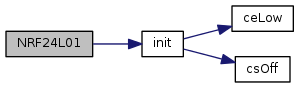## Member Function Documentation

 std::string name ( )
inline

Returns the name of the component

Here is the call graph for this function: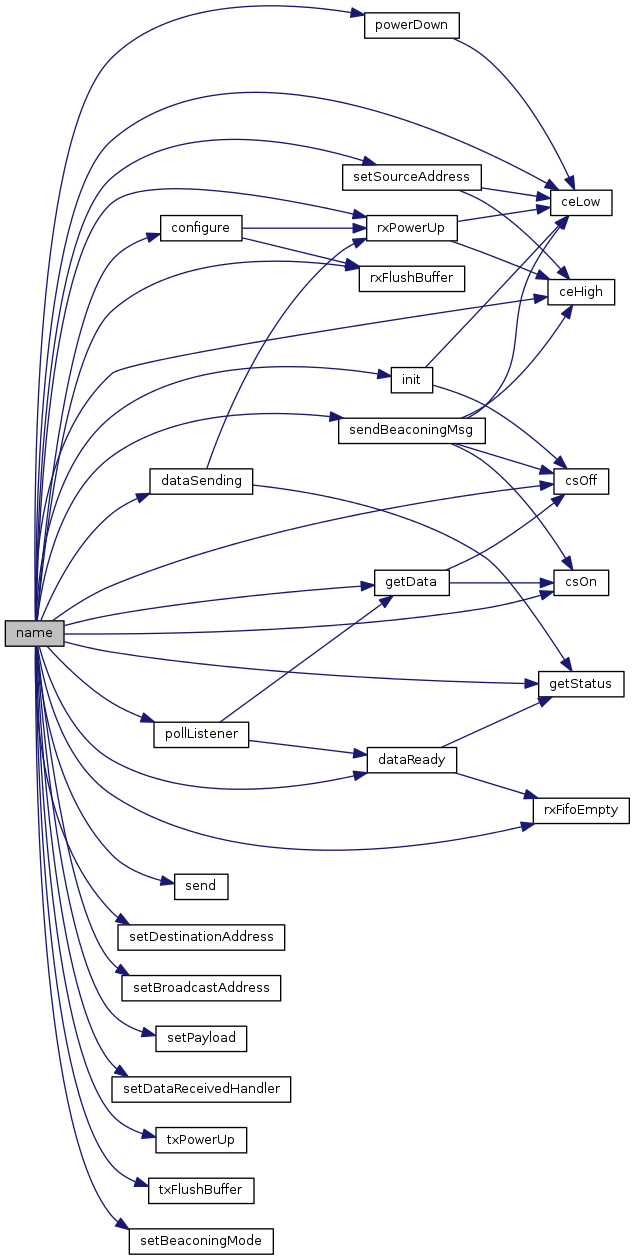void init ( int chipSelect, int chipEnable )

Initializes needed GPIO pins and SPI

Parameters
 chipSelect Sets up the chip select pin chipEnable Sets up the chip enable pin

Here is the call graph for this function: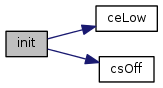Here is the caller graph for this function: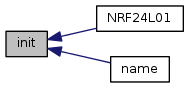void configure ( )

Configures the NRF24L01 transceiver

Here is the call graph for this function: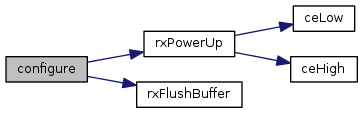Here is the caller graph for this function: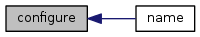void send ( uint8_t * value )

Sends the buffer data

Parameters
 value Pointer to the buffer

Here is the call graph for this function: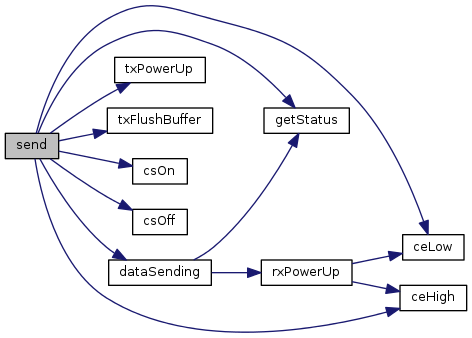void send ( )

Sends the data located in an inner bufer; the user must fill the m_txBuffer buffer

Here is the caller graph for this function: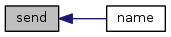void setSourceAddress ( uint8_t * addr )

Sets a receiving address of the device

Parameters

Here is the call graph for this function: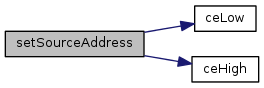Here is the caller graph for this function: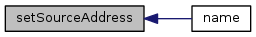void setDestinationAddress ( uint8_t * addr )

Sets a recipient address. The nrfSend method sends the data buffer to this address

Parameters

Here is the caller graph for this function: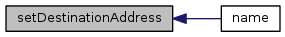Parameters

Here is the caller graph for this function: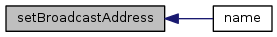Sets the payload size

Parameters
 load Size of the payload (MAX 32)

Here is the caller graph for this function: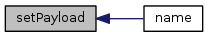void setDataReceivedHandler ( Callback * call_obj )

Sets the handler to be called when data has been received

Parameters
 call_obj Object used for callback - Java

Here is the caller graph for this function: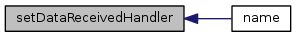bool dataReady ( )

Checks if the data has arrived

Here is the call graph for this function: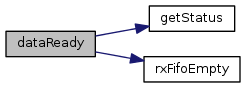Here is the caller graph for this function: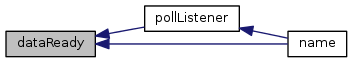bool dataSending ( )

Checks if the transceiver is in the sending mode

Here is the call graph for this function: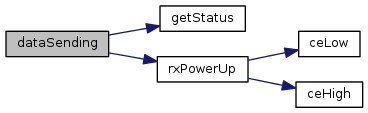Here is the caller graph for this function: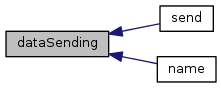void getData ( uint8_t * data )

Sinks all the arrived data into a provided buffer

Parameters
 data Pointer to the buffer

Here is the call graph for this function: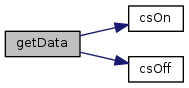Here is the caller graph for this function: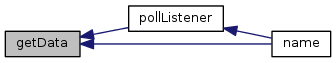uint8_t getStatus ( void )

Checks the transceiver state

Here is the caller graph for this function: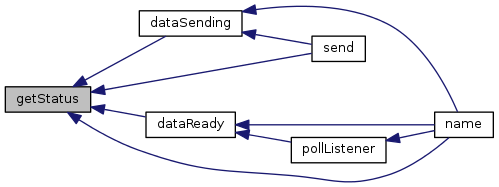bool rxFifoEmpty ( )

Checks if the receive stack is empty

Here is the caller graph for this function: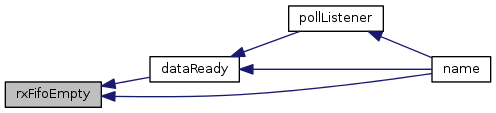void rxPowerUp ( )

Powers the receiver up

Here is the call graph for this function: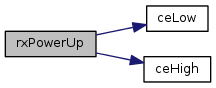Here is the caller graph for this function: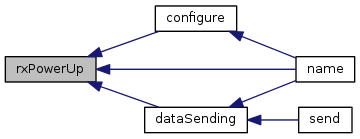void rxFlushBuffer ( )

Flushes the receive stack

Here is the caller graph for this function: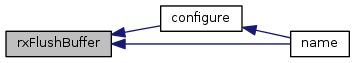void txPowerUp ( )

Powers the transmitter up

Here is the caller graph for this function: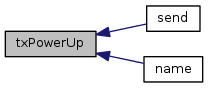void powerDown ( )

Powers everything down

Here is the call graph for this function: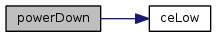Here is the caller graph for this function: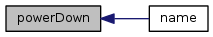void txFlushBuffer ( )

Flushes the transmit stack

Here is the caller graph for this function: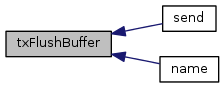void pollListener ( )

Pulling the method listening for the arrived data, dataRecievedHandler is triggered if data arrives

Here is the call graph for this function: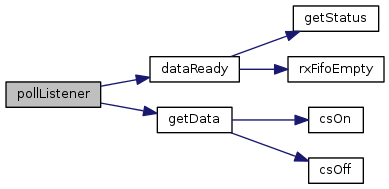Here is the caller graph for this function: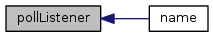mraa::Result ceHigh ( )

Sets the chip enable pin to HIGH

Here is the caller graph for this function: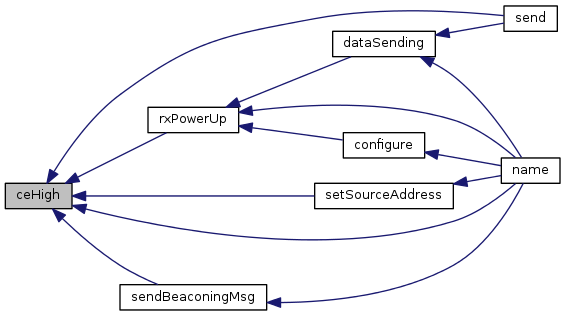mraa::Result ceLow ( )

Sets the chip enable pin to LOW

Here is the caller graph for this function: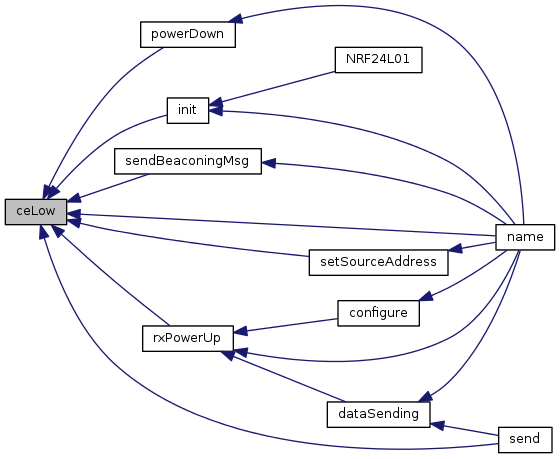mraa::Result csOn ( )

Sets the chip select pin to LOW

Here is the caller graph for this function: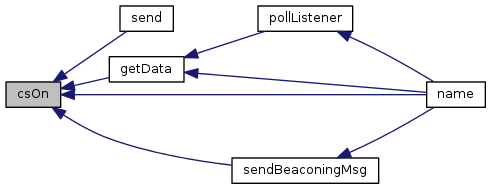mraa::Result csOff ( )

Sets the chip select pin to HIGH

Here is the caller graph for this function: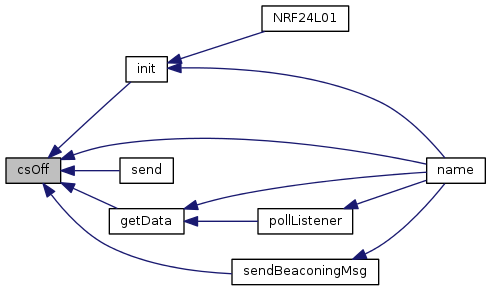void setBeaconingMode ( )

Configures the NRF24L01 transceiver to behave as a BLE (Bluetooth Low Energy) beaconing devcie.

Here is the caller graph for this function: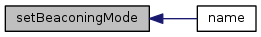void sendBeaconingMsg ( uint8_t * msg )

Beacons the provided message to BLE scanners.

Parameters
 msg Beacons the provided message (max length is 16 bytes)

Here is the call graph for this function: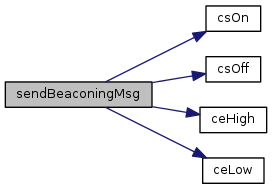Here is the caller graph for this function: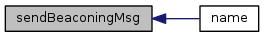## Field Documentation

 uint8_t m_rxBuffer[MAX_BUFFER]